# Use of accessory plates

### 2. Quarter wave plate

The quarter wave plate is a small section of mica with a retardation exactly equal to 1/4 of the retardation of the wave plate examined in preceding section. It has thus no minimum interference in the visible region of the spectrum and gives a light gray color when examined at 45° between crossed polars. The addition of a quarter wave plate to an optic normal conoscopic figure gives the image in figure 2. To explain this image, we can follow the same reasoning developed for the wave plate figure. As calcite is a negative crystal, there is a subtraction of the retardations of crystal and accessory plate along diagonal AB. At the center, both indices of the crystal are equal to the ordinary index w so the retardation is equal to the quarter wave plate alone and gives thus a gray color. Moving from the center along diagonal AB, the retardation decreases and must be zero for a point where retardation of crystal and retardation of 1/4 wave plate are equals. This point is marked  x in figure 2. The position of this point is closer to the center if the birefringence of crystal is higher. Along AB, the retardation is a quarter wave lower than the one observed in figure 1 so the isochromes rings  are moving away from center. On the other diagonal, the retardations of crystal and accessory plate are summed up so no dark region is found. The global retardation along that diagonal is higher by a quarter wave than retardation measured at the same place in figure 1. The rings are consequently closer to the center because the retardation to produce an extinction for a particular wavelength is reached for a lower thickness of crystal so at a lower angle. The difference in retardations between both diagonals is just a half wave so the rings in one direction are just positioned half way between the rings of the other direction. The isogyres take the gray color of the quarter wave plate.

If the crystal is positive, figure 2 should be rotated by 90°. The position of the black spots (x) is characteristic of the optical sign of a crystal and can be used for its determination if the birefringence of the crystal is high enough so the spots are not located outside the field of view.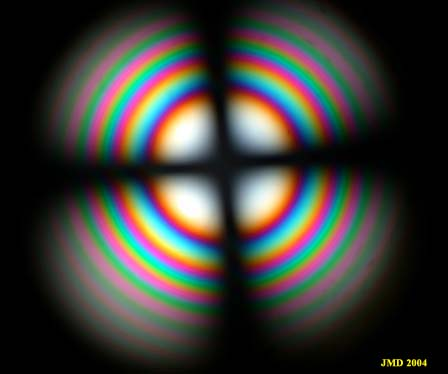Figure 1 . Calcite section perpendicular to optic axis.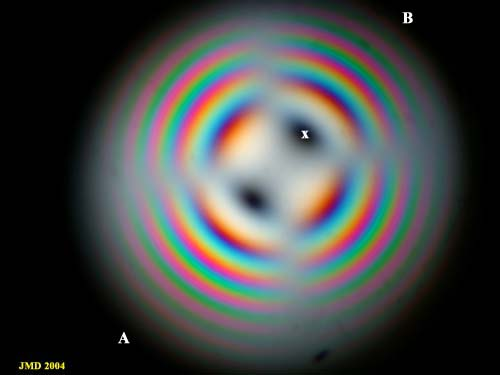Figure 2. Addition of a quarter wave plate.

### 3. The Benford plate.

The Benford plate is not a single plate but the addition of two quarter wave plates, one inserted in the microscope tube at the usual place and the second one positioned below the examined object, oriented perpendicularly to the first quarter wave plate. The two plates are thus at 45 ° from polarizer and analyzer directions.

1. Let us first examine what happens when a Benford plate is installed and no crystal object is present on the microscope stage. The light coming trough the polarizer is resolved by the first quarter wave plate into two vibrations at 45° from polarizer direction. A quarter wave retardation is introduced between the two waves. The second plate being perpendicular to the first one, is in a position of subtraction of the retardations. It subtract thus its own retardation from the retardation introduced by the first plate. As these two plates are identical the result of the subtraction is zero and the field of view is dark. The presence of the Benford plate does not change anything if isotropic material are examined: they remain dark.

2. We place now on the microscope stage an anisotropic crystal section with indices directions at 45° from polarizer priviledged direction. As its indices directions are parallel to the quarter wave plates indices, the effect is an addition of the retardations. As explained in previous section, the two quarter wave plates cancel their retardations so the field of view exhibits the crystal interference color as if no quarter wave plates were present.

3.  We consider now the case of  a non isotropic crystal with its indices directions parallel to polarizer and analyzer priviledged directions. If no Benford plate is present, the light coming through the polarizer pass through the crystal with its polarization plane unchanged because of the plate orientation. This light cannot pass through the crossed analyzer. When the Benford plate is introduced, the light waves entering our specimen crystal have now directions along the first quarter wave plates indices at 45° from the polarizer. As the polarization plane direction has been changed, some light components can pass through the analyzer. As the two quarter wave retardations cancel, only the crystal retardation produces interference colors, so again, the result is the color of the crystal alone. In fact, the Benford plate erases the effect of extinction of the crossed polars showing only interference colors due to waves retardation.

It can be used in orthoscopic examination of a thin section to image in a single view the colors of crystals due to birefringence and thickness. An example is given in figure 3.

In conoscopic examination, the Benford plate erases the isogyres according to the explanation of section 3 but leaves a dark spot at the position of the optic axis as explained in paragraph 1 because the section in indicatrix perpendicular to optic axis is always isotropic (circular section). An example is given in figure 5 below.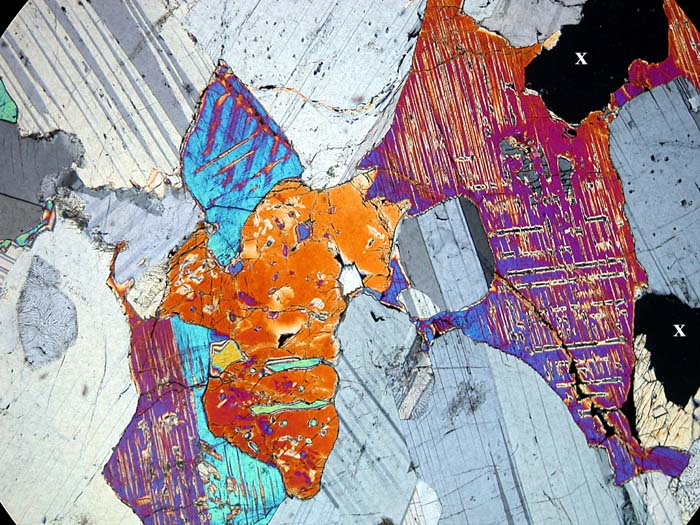Figure 3. View of a Gabbro thin section with a Benford plate. All crystals show their 45° interference color without extinction. The dark region marked X is a hole in the preparation.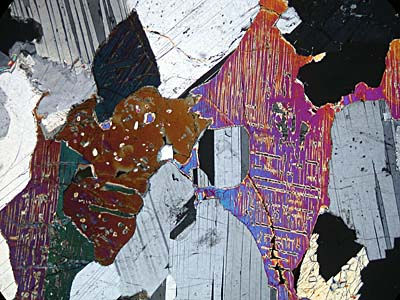Figure 4A. Same Gabbro section between crossed polars showing some extinctions.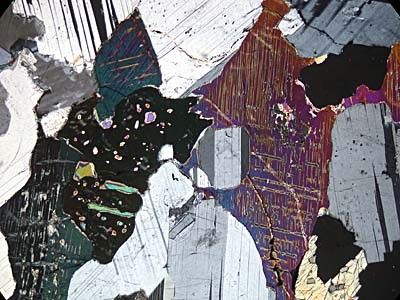Figure 4 B. Same section with other orientation showing other crystals in extinction.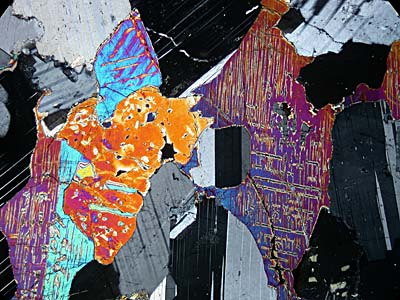Figure 4C. Same section. Other orientation.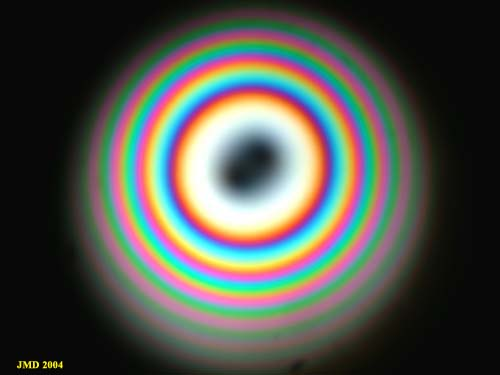Figure 5. Calcite perpendicular to the optic axis with Benford plate. The isogyres disappear, only the pole of the optic axis is dark.

### 4. Quartz wedge

The quartz wedge is an accessory plate whose thickness is increasing along small index.  The large index has the same orientation as wave plate or quarter wave plate. It can be introduced in the slot of the microscope tube and its thickness magnitude can be varied by pushing or withdrawing it.

In white light, the variation of the conoscopic image of calcite as a function of the thickness of the accessory plate can be examined in the following  Quartz Wedge movie  (0.5MB). You must have quick time installed to see the movies. On  the AB diagonal (NE-SW), there is a subtraction of the retardations of the wedge and the negative calcite crystal as explained in the wave plate and quarter wave plate sections. If the thickness of the wedge is increased, a longer light path in the calcite crystal is necessary to compensate for the larger retardation of the quartz wedge thus the effect is a motion of the dark ring towards periphery of the figure. On the other diagonal (NW-SE), we have an addition of the retardations of crystal and quartz wedge, so, if the thickness of the wedge is increased, the overall retardation increases too and one particular interference color is positioned at a lower light path thus closer to the center. The interference rings are moving towards the center of the figure in the NW-SE quadrant.  For a positive crystal, the retardations subtraction occurs in the NW-SE quadrant so rings are moving outside. The effect is just the opposite.

The same phenomenon can also be examined in monochromatic light on the next movie (0.5 MB).